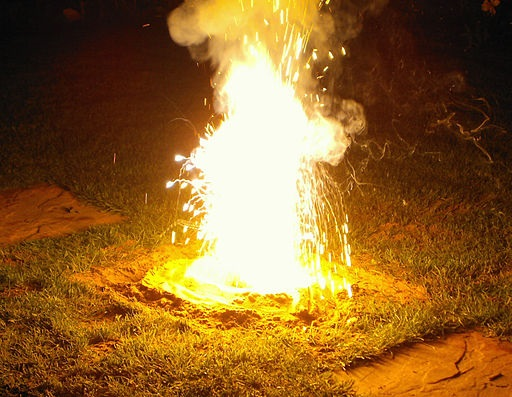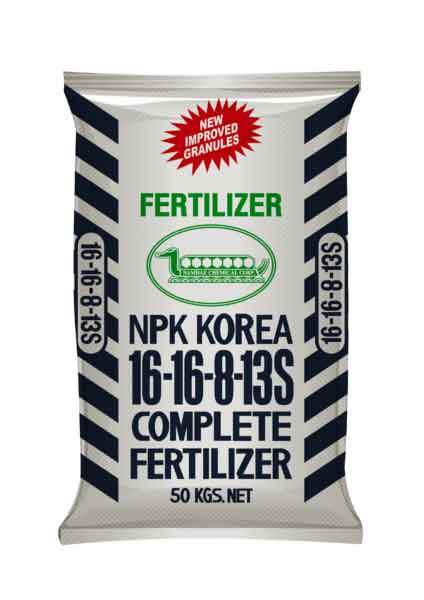Chemistry CIE iGCSE:
Calculations 1a

For Double and Triple sciences

Grab a calculator and a copy of the periodic table

(This test has only 10 questions, but may take 15 - 20 mins).

This test mainly covers calculations involving reacting masses

1. Calculate the relative formula mass (Mr) of calcium hydroxide, Ca(OH)2.
• A.   38
• B.   58
• C.   74
• D.   114
2. Which of the following contains the greatest number of moles?
• A.   2.4 g of carbon, C
• B.   2.3 g of sodium, Na
• C.   2.4 g of Magnesium, Mg
• D.   3.9 g of potassium, K
3. A student required 0.15 moles of magnesium oxide, MgO, for a reaction. What mass of MgO should she weigh out? [Mr of MgO is 40]
• A.   0.00375 g
• B.   0.0060 g
• C    6.0 g
• D.   267 g
4. 2.54g of copper reacts with 0.32g of oxygen gas to produce copper oxide.

A possible balanced equation for this reaction is ...

[Relative atomic masses: Cu = 63.5; O=16]

• A. Cu  +  O  CuO
• B. 2Cu  + O2  2CuO
• C. 4Cu  + O2  2Cu2O
• D. 8Cu  + O2  2Cu4O
5. 60.0g of calcium carbonate decomposed on heating to form calcium oxide and carbon dioxide.   The equation for the reaction is:

CaCO3  CaO + CO2

What is the maximum mass of calcium oxide which could be formed in this reaction?
[Mr values: CaCO3 = 100; CaO = 56; CO2 = 44]

• A.   26.4 g
• B.   30.0 g
• C.   33.6 g
• D.   56.0 g
6. What is a limiting reactant?
• A.   The reactant with the lowest molecular mass
• B.   The reactant that is used in the smallest mass in the reaction
• C.   The reactant that is all used up in the reaction
• D.   The reactant that is left over at the end of a reaction
7. 3g of magnesium was reacted with 0.200 moles of ethanoic acid in the following reaction:

Mg + 2CH3COOH  (CH3COO)2Mg +  H2

Calculate the number of moles of magnesium used and determine the limiting reactant.

 Number of moles of magnesium Reagent in excess A 0.125 Ethanoic acid B 0.125 magnesium C 0.1 Ethanoic acid D 0.1 magnesium
8. In the thermite reaction aluminium powder reacts very exothermically with iron(III)oxide displacing molten iron. The equation for the reaction is:

Fe2O3 + 2Al  2Fe + Al2O3

What mass of molten iron is formed from 0.1moles of iron(III)oxide?
[Mr of Fe2O3 is 160; Ar of Fe is 56]• A.   2.8g
• B.   5.6g
• C.   11.2g
• D.   22.4g

Q9-10: 34.0 kg of ammonia, NH3 is reacted with excess nitric acid, HNO3, to produce ammonium nitrate fertilizer. The equation for the reaction is:

NH3  + HNO3  NH4NO39. Calculate the number of moles of ammonia, NH3, used in this reaction.

[Relative atomic masses H = 1; N = 14, O = 16]

• A.   2.27
• B.   2.00
• C.   2267
• D.   2000
10. Calculate the maximum mass, in kg, of ammonium nitrate formed [Mr of NH4NO3 is 80]
• A.   181.6 kg
• B.   160 kg
• C.   181,600 kg
• D.   160,000 kg